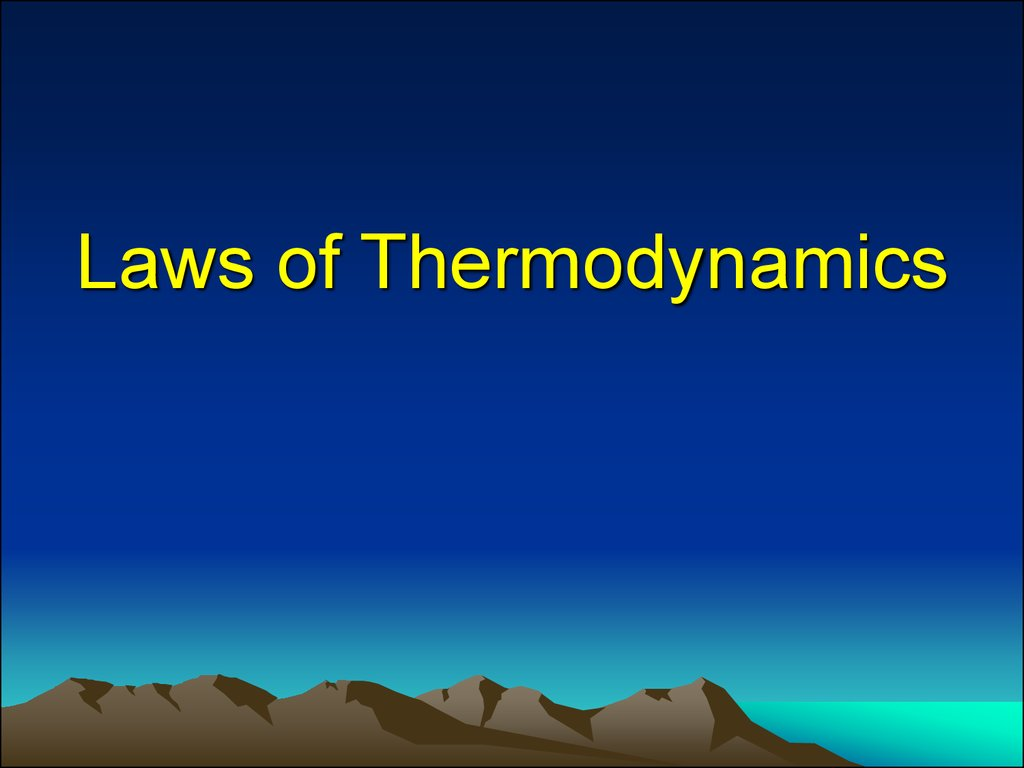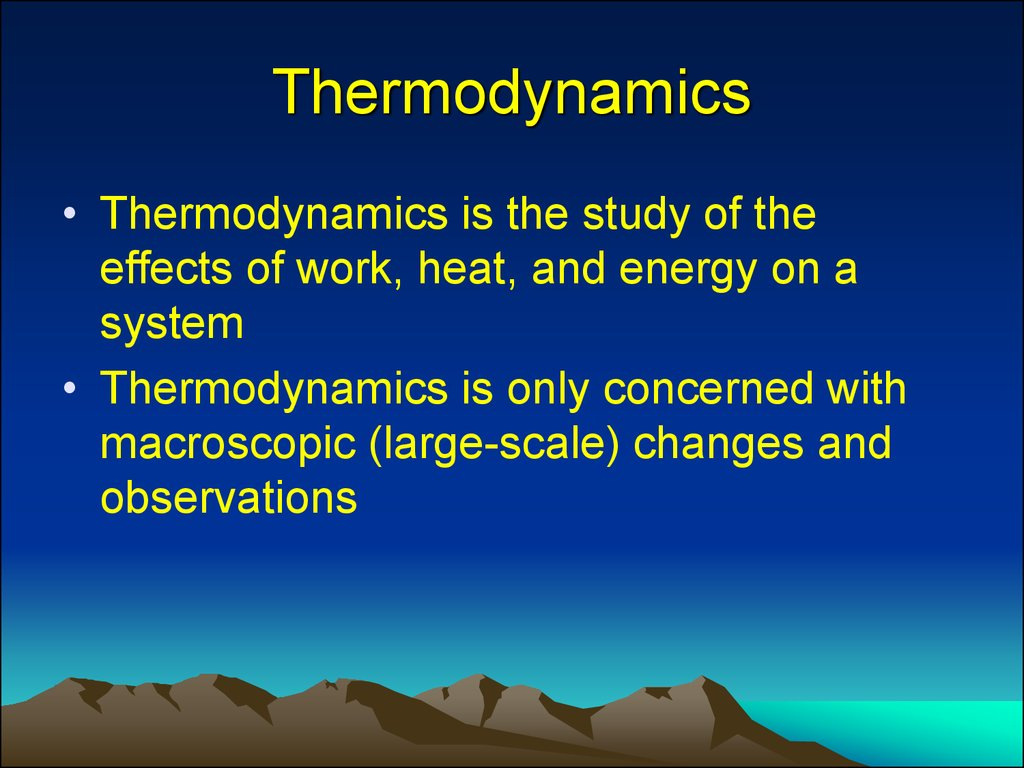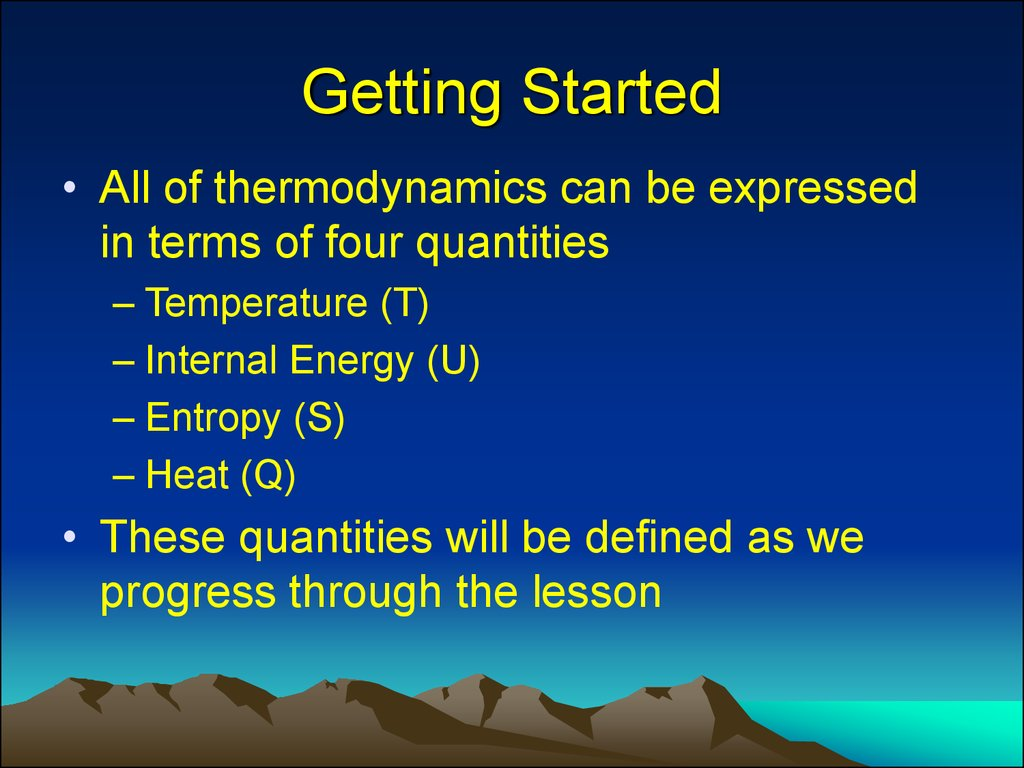# Laws of Thermodynamics

## 2. Thermodynamics

• Thermodynamics is the study of the
effects of work, heat, and energy on a
system
• Thermodynamics is only concerned with
macroscopic (large-scale) changes and
observations

## 3. Getting Started

• All of thermodynamics can be expressed
in terms of four quantities
– Temperature (T)
– Internal Energy (U)
– Entropy (S)
– Heat (Q)
• These quantities will be defined as we
progress through the lesson

## 4. Classical vs Statistical

• Classical thermodynamics concerns the
relationships between bulk properties of
matter. Nothing is examined at the atomic
or molecular level.
• Statistical thermodynamics seeks to
explain those bulk properties in terms of
constituent atoms. The statistical part
treats the aggregation of atoms, not the
behavior of any individual atom

## 5. Introduction

According to British scientist C. P. Snow,
the three laws of thermodynamics can be
(humorously) summarized as
1. You can’t win
2. You can’t even break even
3. You can’t get out of the game

## 6. 1.0 You can’t win (1st law)

• The first law of thermodynamics is an
extension of the law of conservation of
energy
• The change in internal energy of a system
is equal to the heat added to the system
minus the work done by the system
ΔU = Q - W

## 7.

Slide courtesy of NASA

## 8. 1.1 Process Terminology

Isothermal – constant temperature
Isobaric – constant pressure
Isochoric – constant volume

• An adiabatic process transfers no heat
– therefore Q = 0
• ΔU = Q – W
• When a system expands adiabatically, W
is positive (the system does work) so ΔU is
negative.
• When a system compresses adiabatically,
W is negative (work is done on the
system) so ΔU is positive.

## 10. 1.1.2 Isothermal Process

• An isothermal process is a constant
temperature process. Any heat flow into or
out of the system must be slow enough to
maintain thermal equilibrium
• For ideal gases, if ΔT is zero, ΔU = 0
• Therefore, Q = W
– Any energy entering the system (Q) must
leave as work (W)

## 11. 1.1.3 Isobaric Process

• An isobaric process is a constant pressure
process. ΔU, W, and Q are generally nonzero, but calculating the work done by an
ideal gas is straightforward
W = P·ΔV
• Water boiling in a saucepan is an example
of an isobar process

## 12. 1.1.4 Isochoric Process

• An isochoric process is a constant volume
process. When the volume of a system
doesn’t change, it will do no work on its
surroundings. W = 0
ΔU = Q
• Heating gas in a closed container is an
isochoric process

## 13. 1.2 Heat Capacity

• The amount of heat required to raise a
certain mass of a material by a certain
temperature is called heat capacity
Q = mcxΔT
• The constant cx is called the specific heat
of substance x, (SI units of J/kg·K)

## 14. 1.2.1 Heat Capacity of Ideal Gas

• CV = heat capacity at constant volume
CV = 3/2 R
• CP = heat capacity at constant pressure
CP = 5/2 R
• For constant volume
Q = nCVΔT = ΔU
• The universal gas constant R = 8.314 J/mol·K

## 15. 2.0 You can’t break even (2nd Law)

• Think about what it means to not “break
even”. Every effort you put forth, no matter
how efficient you are, will have a tiny bit of
waste.
• The 2nd Law can also be stated that heat
flows spontaneously from a hot object to a
cold object (spontaneously means without the assistance of
external work)

## 16.

Slide courtesy of NASA

## 17. 2.1 Concerning the 2nd Law

• The second law of thermodynamics
introduces the notion of entropy (S), a
measure of system disorder (messiness)
• U is the quantity of a system’s energy, S is
the quality of a system’s energy.
• Another C.P. Snow expression:
– not knowing the 2nd law of thermodynamics is the cultural
equivalent to never having read Shakespeare

## 18. 2.2 Implications of the 2nd Law

• Time marches on
– If you watch a movie, how do you know that
you are seeing events in the order they
occurred?
– If I drop a raw egg on the floor, it becomes
extremely “disordered” (greater Entropy) –
playing the movie in reverse would show
pieces coming together to form a whole egg
(decreasing Entropy) – highly unlikely!

## 19. 2.3 Direction of a Process

• The 2nd Law helps determine the preferred
direction of a process
• A reversible process is one which can
original state
• This is an idealized condition – all real
processes are irreversible

## 20. 2.4 Heat Engine

• A device which transforms heat into work
is called a heat engine
• This happens in a cyclic process
• Heat engines require a hot reservoir to
supply energy (QH) and a cold reservoir to
take in the excess energy (QC)
– QH is defined as positive, QC is negative

## 21. 2.4.1 Cycles

• It is beyond the scope of this presentation,
but here would be a good place to
elaborate on:
– Otto Cycle
– Diesel Cycle
– Carnot Cycle
• Avoid all irreversible processes while adhering to
the 2nd Law (isothermal and adiabatic only)

## 22. 2.4.2 The Carnot Cycle

Image from Keta - Wikipedia

## 23. 2.4.2.1 Carnot explained

• Curve A (1 → 2): Isothermal expansion at TH
– Work done by the gas
• Curve B (2 → 3): Adiabatic expansion
– Work done by the gas
• Curve C (3 → 4): Isothermal compression at TC
– Work done on the gas
• Curve D (4 → 1): Adiabatic compression
– Work done on the gas

## 24. 2.4.2.2 Area under PV curve

• The area under the PV curve represents
the quantity of work done in a cycle
• When the curve goes right to left, the work
is negative
• The area enclosed by the four curves
represents the net work done by the
engine in one cycle

## 25. 2.5 Engine Efficiency

• The thermal efficiency of a heat engine is
e = 1 + QC/QH
• The “engine” statement of the 2nd Law:
– it is impossible for any system to have an
efficiency of 100% (e = 1) [Kelvin’s statement]
• Another statement of the 2nd Law:
– It is impossible for any process to have as its
sole result the transfer of heat from a cooler
object to a warmer object [Clausius’s statement]

## 26. 2.6 Practical Uses

• Automobile engines, refrigerators, and air
conditioners all work on the principles laid
out by the 2nd Law of Thermodynamics
• Ever wonder why you can’t cool your
kitchen in the hot summer by leaving the
refrigerator door open?
– Feel the air coming off the back - you heat the
air outside to cool the air inside
– See, you can’t break even!

## 27. 3.0 You can’t get out (3rd Law)

• No system can reach absolute zero
• This is one reason we use the Kelvin
temperature scale. Not only is the internal
energy proportional to temperature, but
you never have to worry about dividing by
zero in an equation!
• There is no formula associated with
the 3rd Law of Thermodynamics

## 28. 3.1 Implications of 3rd Law

• MIT researchers achieved 450 picokelvin
in 2003 (less than ½ of one billionth!)
• Molecules near these temperatures have
been called the fifth state of matter:
Bose-Einstein Condensates
– Awesome things like super-fluidity and superconductivity happen at these temperatures
– Exciting frontier of research

## 29. 4.0 The Zeroth Law

• The First and Second Laws were well
entrenched when an additional Law was
recognized (couldn’t renumber the 1st and 2nd Laws)
• If objects A and B are each in thermal
equilibrium with object C, then A and B are
in thermal equilibrium with each other
• Allows us to define temperature relative to
an established standard

## 30.

Slide courtesy of NASA

## 31. 4.1 Temperature Standards

• See Heat versus Temperature slides for a
discussion of these two concepts, and the
misconceptions surrounding them
– Heat is energy transfer
– Temperature is proportional to internal energy
– Fahrenheit, Celsius, and Kelvin temp scales